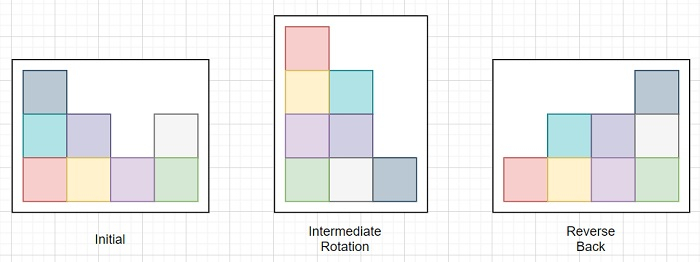# C++ Program to get blocks position after right side rotation

Suppose we have an array A with n elements. A[i] represents there are A[i] number of blocks stacked on top of each other at ith column. The entire blocks are inside a closed transparent boundary box. Now if we rotate the entire big box clockwise 90°, then due to change of gravity direction, the blocks will fall, after than reverse it to its previous orientation. Then find the new array like A after these operations.

## Problem Category

This problem falls under sorting problems. Sorting is a very common problem while we are talking about different problem solving algorithms in computer science. As the name suggests, sorting indicates arranging a set of data into some fashion. We can arrange them in nondecreasing order or non-increasing order in general. Otherwise sorting can be also takes place in a pre-defined manner. For the string based problems, sometimes we refer lexicographical sorting to arrange letters in dictionary fashion. There are plenty of different sorting techniques with certain variations and their time and space complexity. To date, the lower-bound of the time complexity for comparison based sorting techniques is O(n*log n). However there are some mechanical sorting techniques like bucket sort, radix sort, counting sorts are there whose time complexity is linear O(n) in time. For further reading, please follow the link below −

https://www.tutorialspoint.com/data_structures_algorithms/sorting_algorithms.htm

So, if the input of our problem is like A = [3, 2, 1, 2], then the output will be [1, 2, 2, 3]## Steps

To solve this, we will follow these steps −

sort the array A
for initialize i := 0, when i < size of A, update (increase i by 1), do:
print A[i]

## Example

Let us see the following implementation to get better understanding −

#include <bits/stdc++.h>
using namespace std;
void solve(vector<int> A){
sort(A.begin(), A.end());
for (int i = 0; i < A.size(); i++)
cout << A[i] << ", ";
}
int main(){
vector<int> A = { 3, 2, 1, 2 };
solve(A);
}

## Input

{ 3, 2, 1, 2 }

## Output

1, 2, 2, 3,